# Zigzag expanded navigation plots in R: The R package zenplots

#### 2020-11-28

This vignette accompanies the paper “Zigzag expanded navigation plots in R: The R package zenplots”. Note that sections are numbered accordingly (or omitted). Furthermore, it is recommended to read the paper to follow this vignette.

``````# attaching required packages
library(PairViz)
library(MASS)
library(zenplots)``````

## 2 Zenplots

As example data, we use the `olive` data set:

``data(olive, package = "zenplots")``

Reproducing the plots of Figure 1:

``zenplot(olive)``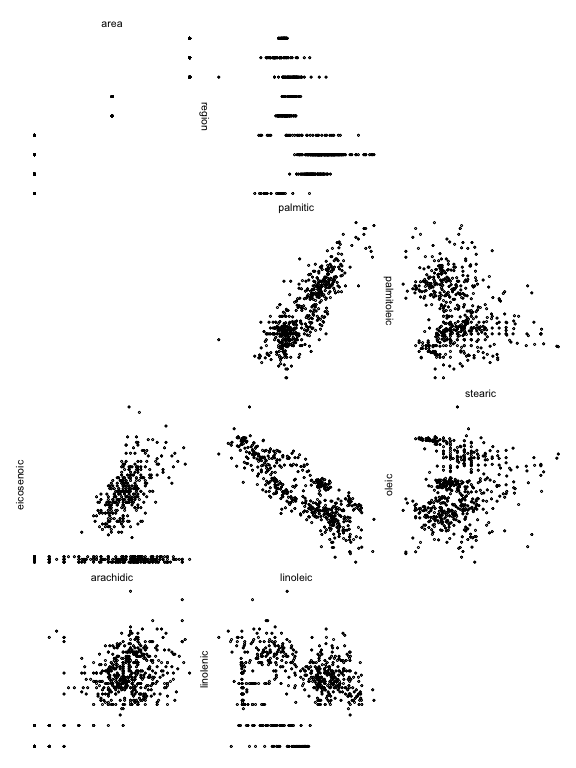``zenplot(olive, plot1d = "layout", plot2d = "layout")``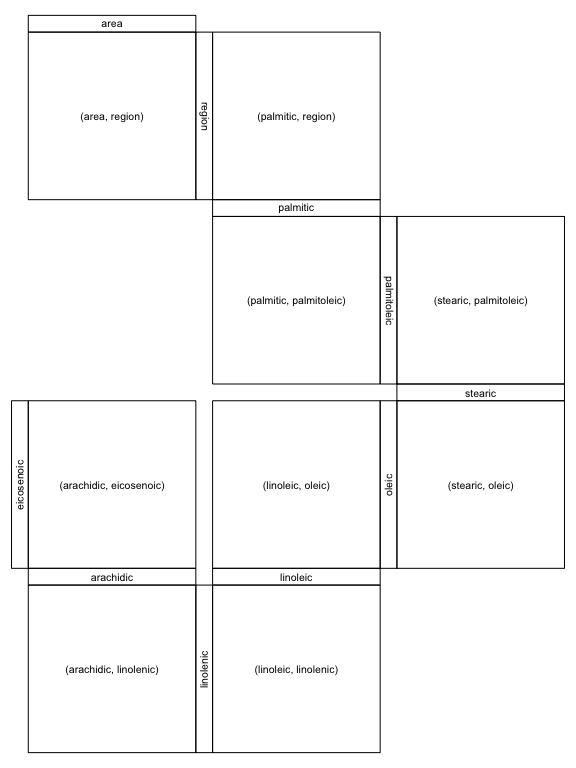Considering the `str()`ucture of `zenplot()` (here formatted for nicer output):

``str(zenplot)``
``````function (x, turns = NULL, first1d = TRUE, last1d = TRUE,
n2dcols = c("letter", "square", "A4", "golden", "legal"),
n2dplots = NULL,
plot1d = c("label", "points", "jitter", "density", "boxplot",
"hist", "rug", "arrow", "rect", "lines", "layout"),
plot2d = c("points", "density", "axes", "label", "arrow",
"rect", "layout"),
zargs = c(x = TRUE, turns = TRUE, orientations = TRUE,
vars = TRUE, num = TRUE, lim = TRUE, labs = TRUE,
width1d = TRUE, width2d = TRUE,
ispace = match.arg(pkg) != "graphics"),
lim = c("individual", "groupwise", "global"),
labs = list(group = "G", var = "V", sep = ", ", group2d = FALSE),
pkg = c("graphics", "grid", "loon"),
method = c("tidy", "double.zigzag", "single.zigzag"),
width1d = if (is.null(plot1d)) 0.5 else 1,
width2d = 10,
ospace = if (pkg == "loon") 0 else 0.02,
ispace = if (pkg == "graphics") 0 else 0.037, draw = TRUE, ...)``````

### 2.1 Layout

To investigate the layout options of zenplots a bit more, we need a larger data set. To this end we simply double the olive data here (obviously only for illustration purposes):

``olive2 <- cbind(olive, olive) # just for this illustration``

Reproducing the plots of Figure 2:

``````zenplot(olive2, n2dcols = 6, plot1d = "layout", plot2d = "layout",
method = "single.zigzag")``````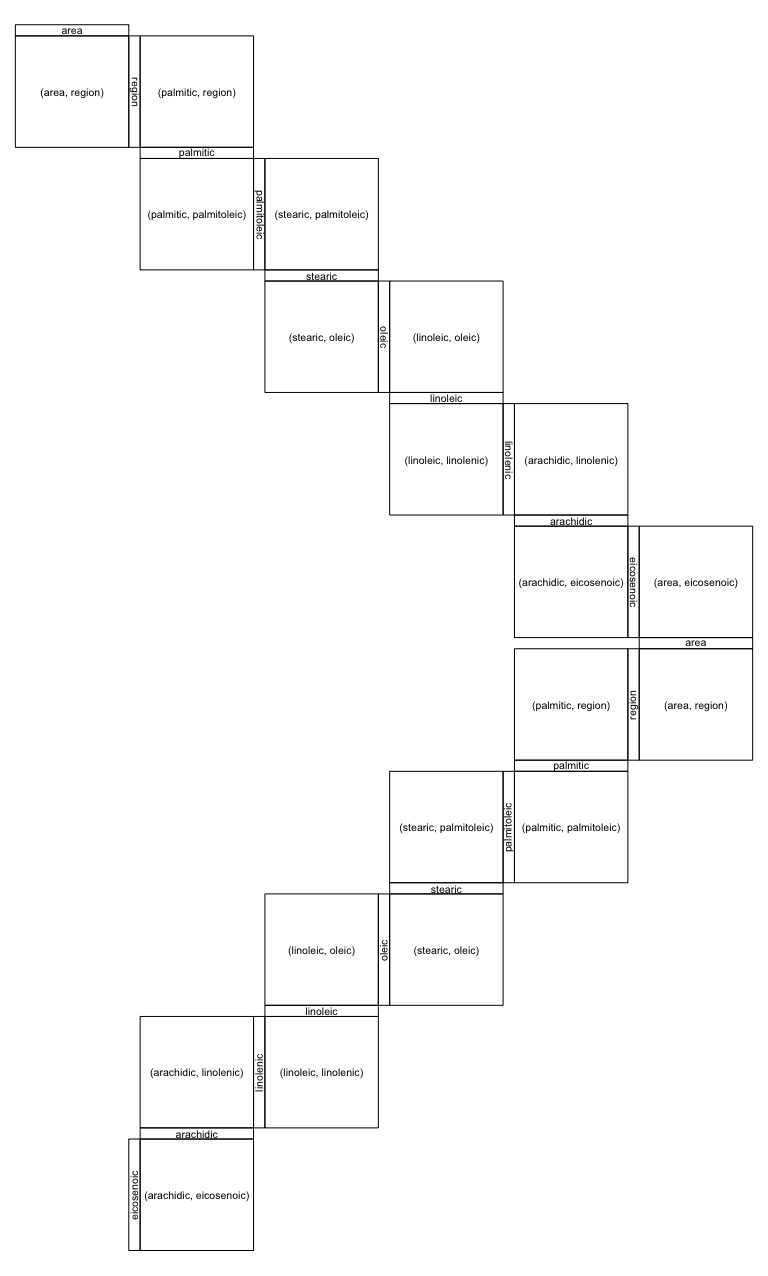``````zenplot(olive2, n2dcols = 6, plot1d = "layout", plot2d = "layout",
method = "double.zigzag")``````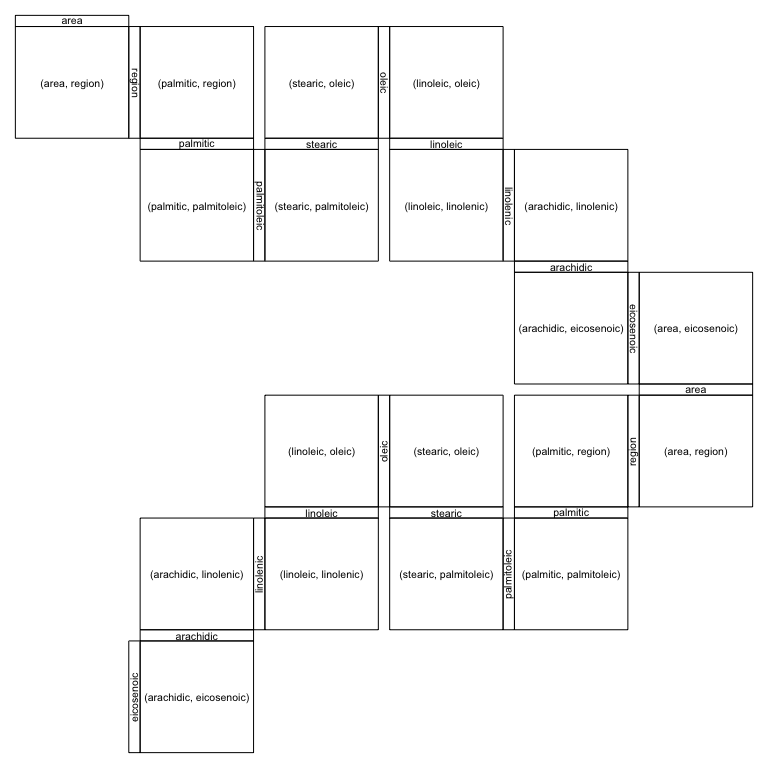``````zenplot(olive2, n2dcols = 6, plot1d = "layout", plot2d = "layout",
method = "tidy")``````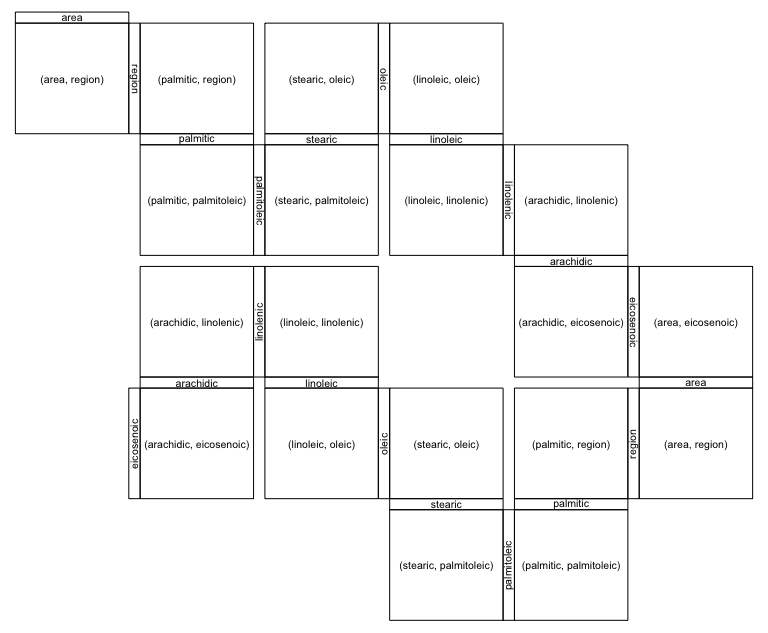Note that there is also `method = "rectangular"` (leaving the zigzagging zenplot paradigm but being useful for laying out 2d plots which are not necessarily connected through a variable; note that in this case, we omit the 1d plots as the default (labels) is rather confusing in this example):

``````zenplot(olive2, n2dcols = 6, plot1d = "arrow", plot2d = "layout",
method = "rectangular")``````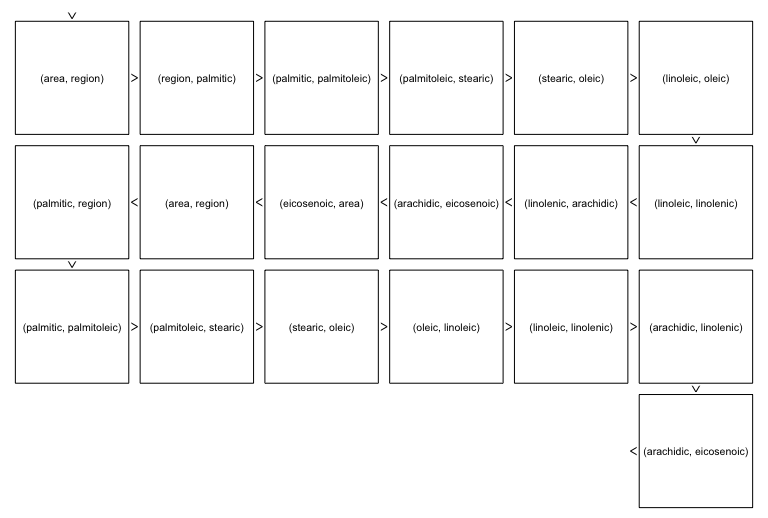Reproducing the plots of Figure 3:

``````zenplot(olive, plot1d = "layout", plot2d = "layout", method = "double.zigzag",
last1d = FALSE, ispace = 0.1)``````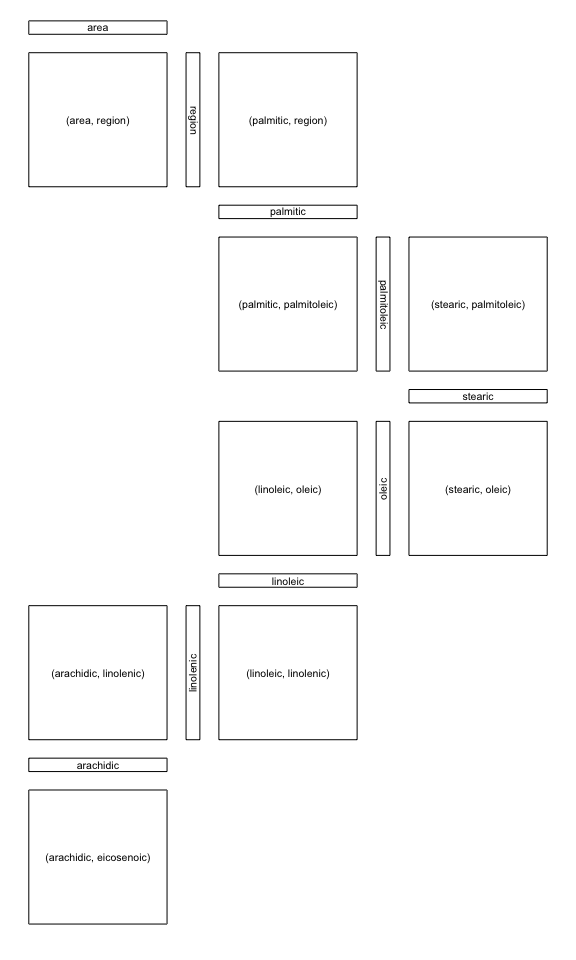``````zenplot(olive, plot1d = "layout", plot2d = "layout", n2dcol = 4, n2dplots = 8,
width1d = 2, width2d = 4)``````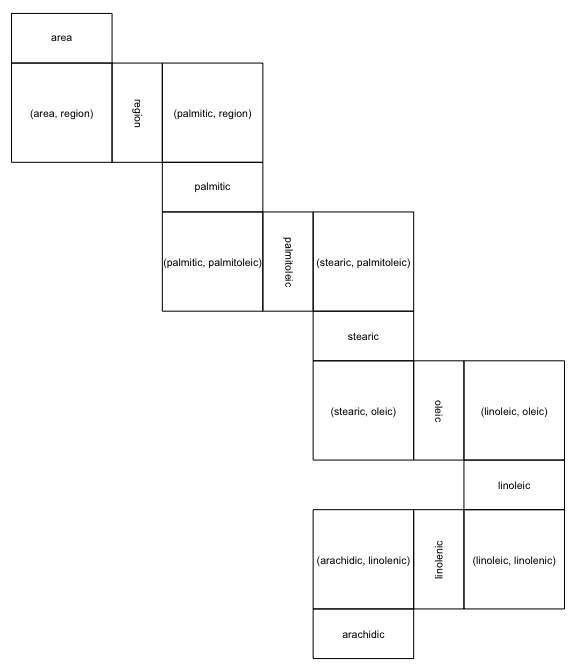## 3 Zenpaths

A very basic path (standing for the sequence of pairs (1,2), (2,3), (3,4), (4,5)):

``(path <- 1:5)``
``##  1 2 3 4 5``

A zenpath through all pairs of variables (Eulerian):

``(path <- zenpath(5))``
``##   5 1 2 3 1 4 2 5 3 4 5``

If `dataMat` is a five-column matrix, the zenplot of all pairs would then be constructed as follows:

``zenplot(x = dataMat[,path])``

The `str()`ucture of `zenpath()` (again formatted for nicer output):

``str(zenpath)``
``````function (x, pairs = NULL,
"eulerian.cross", "greedy.weighted", "strictly.weighted"),
decreasing = TRUE)``````

Here are some methods for five variables:

``zenpath(5, method = "front.loaded")``
``##   5 1 2 3 1 4 2 5 3 4 5``
``zenpath(5, method = "back.loaded")``
``##   1 2 3 1 4 2 5 3 4 5 1``
``zenpath(5, method = "balanced")``
``##   1 2 3 5 4 1 3 4 2 5 1``

The following method considers two groups: One of size three, the other of size five. The sequence of pairs is constructed such that the first variable comes from the first group, the second from the second.

``zenpath(c(3,5), method = "eulerian.cross")``
``##   1 4 2 5 1 6 2 7 1 8 3 4 3 6 7 3 5 8 2``

Reproducing Figure 4:

``````oliveAcids <- olive[, !names(olive) %in% c("area", "region")] # acids only
zpath <- zenpath(ncol(oliveAcids)) # all pairs
zenplot(oliveAcids[, zpath], plot1d = "hist", plot2d = "density")``````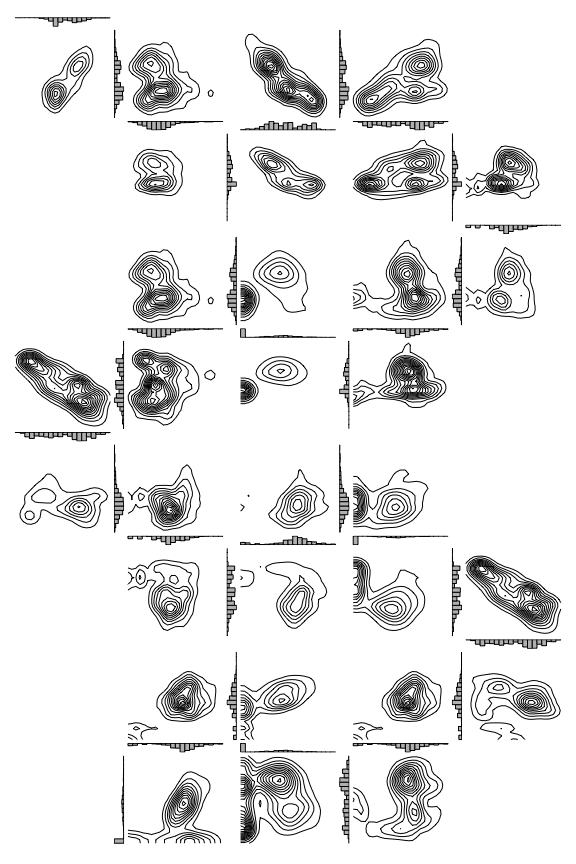## 4 Build your own zenplots

### 4.3 Custom layout and plots – a spiral of ggplots example

Figure 5 can be reproduced as follows (note that we do not show the plot here due to a CRAN issue when running this vignette):

``````path <- c(1,2,3,1,4,2,5,1,6,2,7,1,8,2,3,4,5,3,6,4,7,3,8,4,5,6,7,5,8,6,7,8)
turns <- c("l",
"d","d","r","r","d","d","r","r","u","u","r","r","u","u","r","r",
"u","u","l","l","u","u","l","l","u","u","l","l","d","d","l","l",
"u","u","l","l","d","d","l","l","d","d","l","l","d","d","r","r",
"d","d","r","r","d","d","r","r","d","d","r","r","d","d")

library(ggplot2) # for ggplot2-based 2d plots
stopifnot(packageVersion("ggplot2") >= "2.2.1") # need 2.2.1 or higher
ggplot2d <- function(zargs) {
r <- extract_2d(zargs)
num2d <- zargs\$num/2
df <- data.frame(x = unlist(r\$x), y = unlist(r\$y))
p <- ggplot() +
geom_point(data = df, aes(x = x, y = y), cex = 0.1) +
theme(axis.line = element_blank(),
axis.ticks = element_blank(),
axis.text.x = element_blank(),
axis.text.y = element_blank(),
axis.title.x = element_blank(),
axis.title.y = element_blank())
if(num2d == 1) p <- p +
theme(panel.background = element_rect(fill = 'royalblue3'))
if(num2d == (length(zargs\$turns)-1)/2) p <- p +
theme(panel.background = element_rect(fill = 'maroon3'))
ggplot_gtable(ggplot_build(p))
}

zenplot(as.matrix(oliveAcids)[,path], turns = turns, pkg = "grid",
plot2d = function(zargs) ggplot2d(zargs))``````

### 4.4 Data groups

Split the olive data set into three groups (according to their variable `area`):

``````oliveAcids.by.area <- split(oliveAcids, f = olive\$area)
# Replace the "." by " " in third group's name
names(oliveAcids.by.area) <- gsub("\\.", " ", names(oliveAcids.by.area))
names(oliveAcids.by.area)``````
``##  "South"        "Sardinia"     "Centre North"``

Reproducing the plots of Figure 6 (note that `lim = "groupwise"` does not make much sense here as a plot):

``zenplot(oliveAcids.by.area, labs = list(group = NULL))``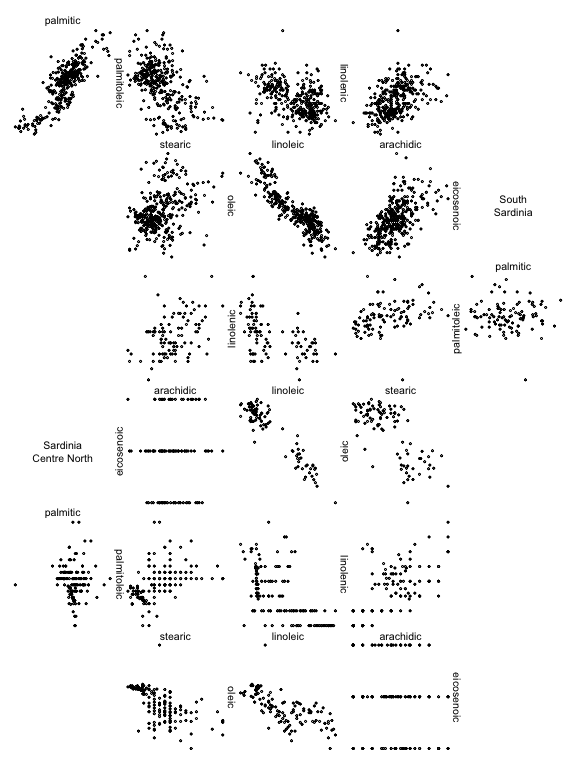``````zenplot(oliveAcids.by.area, lim = "groupwise", labs = list(sep = " - "),
plot1d = function(zargs) label_1d_graphics(zargs, cex = 0.8),
plot2d = function(zargs)
points_2d_graphics(zargs, group... = list(sep = "\n - \n")))``````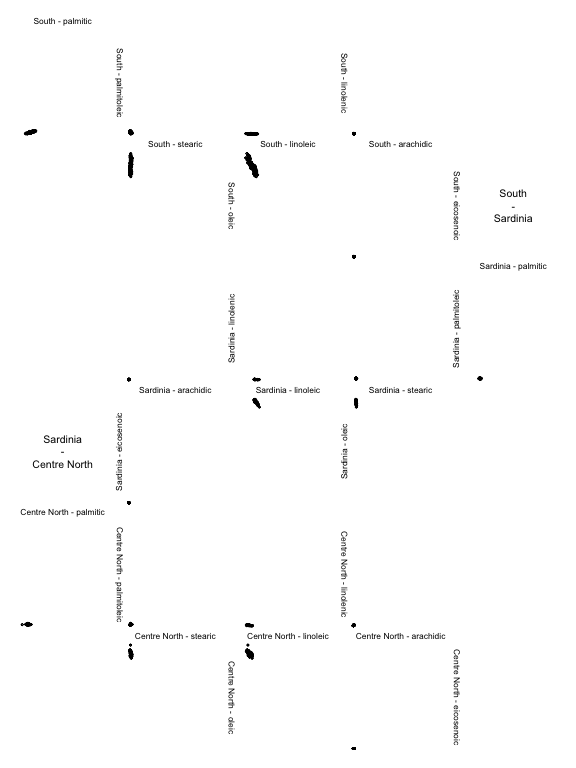### 4.5 Custom zenpaths

Find the “convexity” scagnostic for each pair of olive acids.

``````library(scagnostics)
Y <- scagnostics(oliveAcids) # compute scagnostics (scatter-plot diagonstics)
X <- Y["Convex",] # pick out component 'convex'
d <- ncol(oliveAcids)
M <- matrix(, nrow = d, ncol = d) # matrix with all 'convex' scagnostics
M[upper.tri(M)] <- X # (i,j)th entry = scagnostic of column pair (i,j) of oliveAcids
M[lower.tri(M)] <- t(M)[lower.tri(M)] # symmetrize
round(M, 5)``````
``````##         [,1]    [,2]    [,3]    [,4]    [,5]    [,6]    [,7]    [,8]
## [1,]      NA 0.48952 0.46343 0.45887 0.43914 0.34583 0.31259 0.28413
## [2,] 0.48952      NA 0.42276 0.50499 0.44591 0.35855 0.35846 0.31729
## [3,] 0.46343 0.42276      NA 0.39700 0.36394 0.31316 0.29534 0.33709
## [4,] 0.45887 0.50499 0.39700      NA 0.46454 0.36616 0.29451 0.34888
## [5,] 0.43914 0.44591 0.36394 0.46454      NA 0.31977 0.31443 0.36750
## [6,] 0.34583 0.35855 0.31316 0.36616 0.31977      NA 0.53726 0.34001
## [7,] 0.31259 0.35846 0.29534 0.29451 0.31443 0.53726      NA 0.22231
## [8,] 0.28413 0.31729 0.33709 0.34888 0.36750 0.34001 0.22231      NA``````

Show the six pairs with largest “convexity” scagnostic:

``````zpath <- zenpath(M, method = "strictly.weighted") # list of ordered pairs
head(M[do.call(rbind, zpath)]) # show the largest six 'convexity' measures``````
``##  0.5372599 0.5049945 0.4895179 0.4645377 0.4634277 0.4588675``

Extract the corresponding pairs:

``(ezpath <- extract_pairs(zpath, n = c(6, 0))) # extract the first six pairs``
``````## []
##  7 6
##
## []
##  4 2
##
## []
##  2 1
##
## []
##  5 4
##
## []
##  3 1
##
## []
##  4 1``````

Reproducing Figure 7 (visualizing the pairs):

``````library(graph)
plot(graph_pairs(ezpath)) # depict the six most convex pairs (edge = pair)``````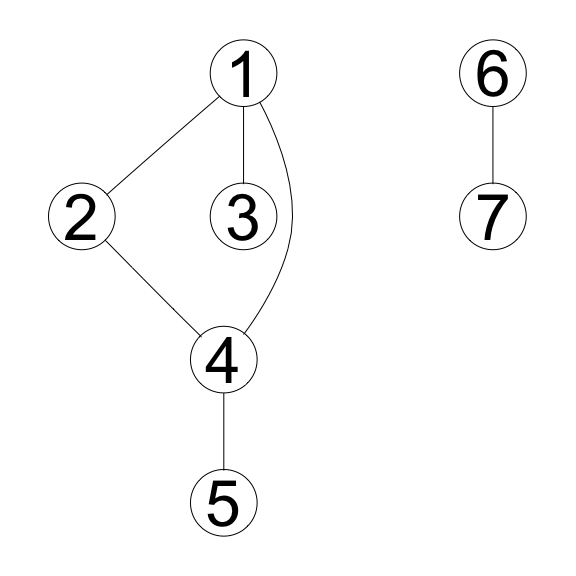Connect them:

``(cezpath <- </``